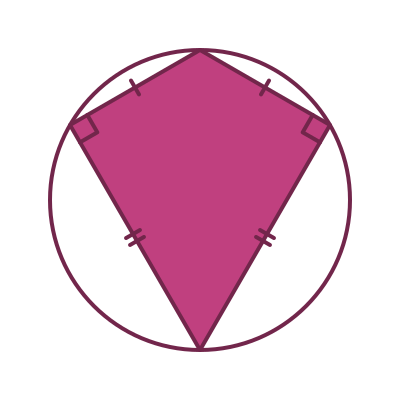By Martin McBride, 2022-11-11
Tags: quadrilateral square rectangle parallelogram rhombus trapezium isosceles trapezium kite
Categories: gcse geometryA quadrilateral is a shape with four straight sides.

There are various types of quadrilaterals that have special names:

• Square
• Rectangle
• Parallelogram
• Rhombus
• Trapezium
• Kite

## Square

A square is a quadrilateral where all four sides are equal, and all four angles are 90 degrees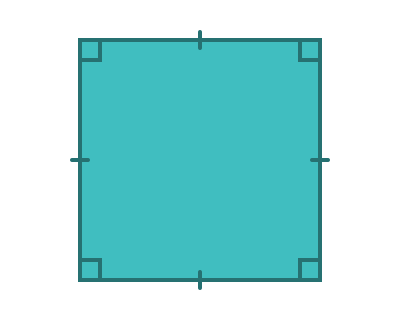The tick marks on the four sides indicate that the sides are the same length.

The square angle symbols in the corners indicate that each corner is a 90-degree angle.

## Rectangle

A rectangle is a quadrilateral that has all four angles equal to 90 degrees.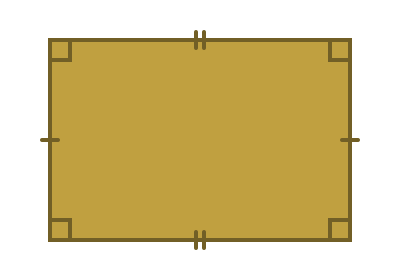The opposite sides of a rectangle are always equal.

A square is a special type of rectangle, where all four sides are equal.

## Parallelogram

A parallelogram is a quadrilateral where both pairs of opposite sides are parallel. You can think of a parallelogram as a "pushed-over" rectangle.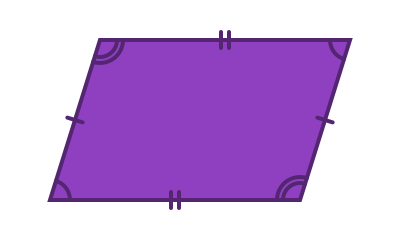The opposite sides of a parallelogram have equal lengths.

The diagonally opposite angles are equal.

A rectangle is a special type of parallelogram where all 4 angles are 90 degrees.

## Rhombus

A rhombus is a quadrilateral where all four sides are the same length, and both pairs of opposite sides are parallel. You can think of a rhombus as a "pushed-over" square.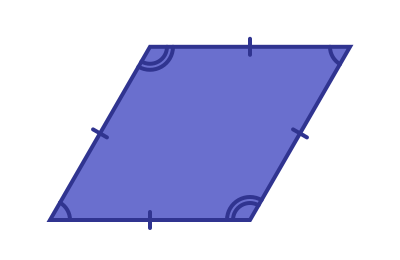A rhombus is a special type of parallelogram where all four sides are equal. The diagonally opposite angles of a rhombus are equal.

If you rotate a rhombus you will see a shape that is sometimes called a diamond. It is the same shape, just rotated.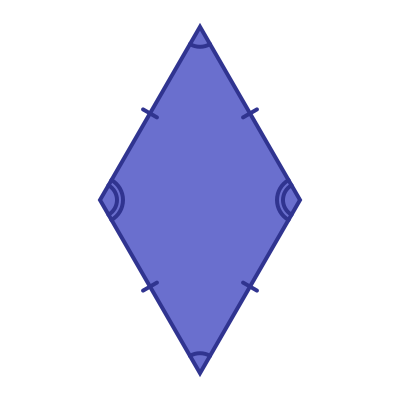## Trapezium

A trapezium is a quadrilateral that has at least two parallel sidesThere are several types of trapezium.

### Acute trapezium

An acute trapezium is a trapezium where both angles at the base are acute (that is, less than 90 degrees). It is like a slice through an acute triangle.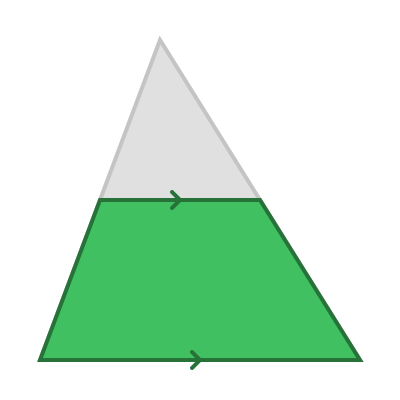Notice that the sides slope in opposite directions.

### Obtuse trapezium

An obtuse trapezium is a trapezium where one of the angles at the base is obtuse (that is, greater than 90 degrees). It is like a slice through an obtuse triangle.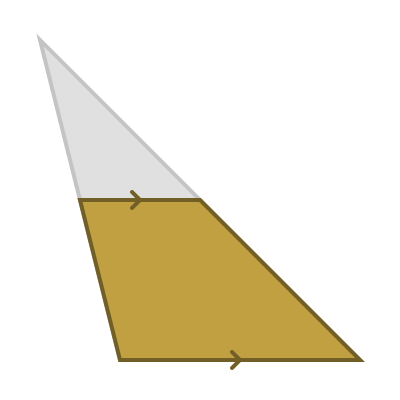Notice that one side slopes inwards, and one slopes outwards.

### Right-angled trapezium

A right-angled trapezium is a trapezium where one of the angles at the base is a right angle. It is like a slice through a right-angled triangle.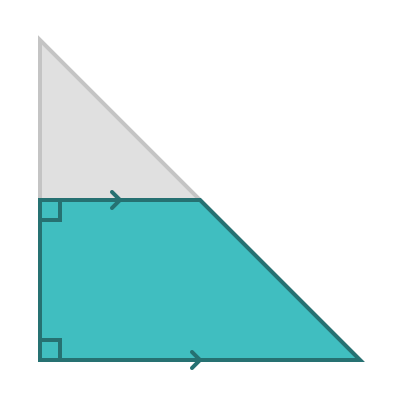### Isosceles trapezium

An isosceles trapezium is one where the two sides make the same angle to the base (but with opposite directions of slope).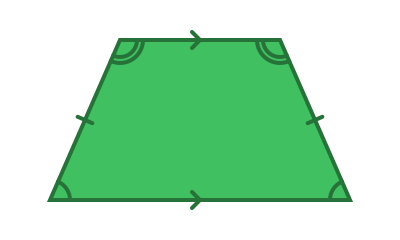The two sides of an isosceles trapezium are of equal length. An isosceles trapezium is like a slice through an isosceles triangle.

### Trapezium with three equal sides

A special case of the isosceles trapezium is one where three of the sides are equal in length: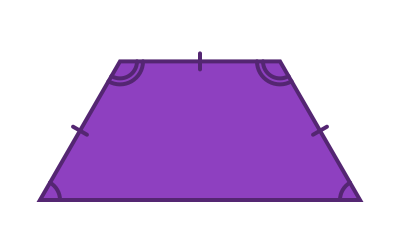## Kite

A kite is a quadrilateral that has two pairs of equal-length sides that are adjacent to each other (that is, next to each other).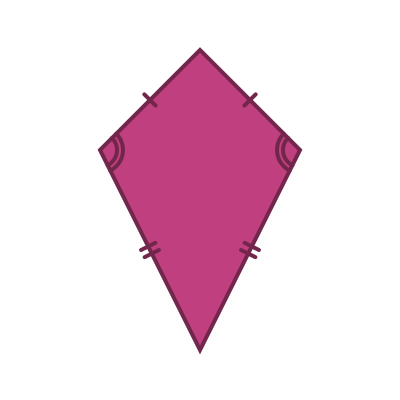The opposite angles of a kite are equal, as shown.

A rhombus is a special case of a kite where the lengths of all four sides are equal.

### Right kite

A kite always has two equal angles - the angles between a short and long side. A right kite is a special case where those angles are right angles.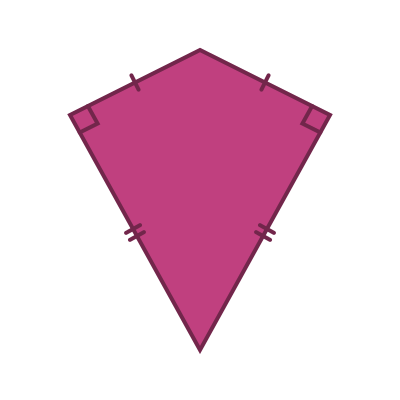An interesting fact about right kites is they are cyclic - you can draw a circle that touches all four corners.